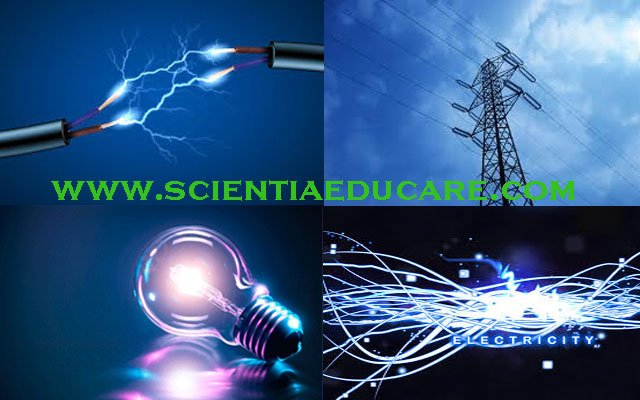Home Education ELECTRICITY QUIZ

# ELECTRICITY QUIZ

1053
0Electricity is the set of physical phenomena associated with the presence and flow of electric charge. Electricity gives a wide variety of well-known effects, such as lightning, static electricity, electromagnetic induction and electrical current.

Electricity Quiz

1. Electric current is measured using what device?
2. True or false? Batteries convert chemical to electrical energy.
3. In terms of electricity, what does DC stand for?
4. The wire inside an electric bulb is known as the what?
5. Conductors have a high or low resistance?
6. True or false? The concept of electric fields was first introduced by Albert Einstein.
7. Electric resistance is typically measured in what units?
8. In terms of electricity, what does AC stand for?
9. Electric power is typically measured in what units?
10. True or false? You can extend battery life by storing batteries at a low temperature.

1. Ammeter
2. True
3. Direct current
4. Filament
5. Low